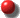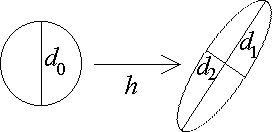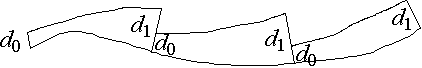# Lyapunov Exponents

## 10/3/00 Lecture #5 in Physics 505

###Comments on Homework #3 (Van der Pol Equation)

• Some people only took initial conditions inside the attractor
• For b < 0 the attractor becomes a repellor (time reverses)
• The driven system can give limit cycles and toruses but not chaos (?)
• Can get chaos if you drive the dx/dt equation instead of dy/dt

###Review (last week) - Dynamical Systems Theory

• Types of attractors/repellors:
• Limit cycles (closed loops) 1-D
• 2-Toruses (quasiperiodic surfaces) 2-D
• N-Toruses (hypersurfaces) N-D
• Strange attractors (fractal) Non-integer D

• (Attractor dimension < system dimension)
• Stability of equilibrium points:
• Find equilibrium point: f(x) = 0  ==> x*, etc.
• Calculate partial derivatives fx etc. at equilibrium
• Construct the Jacobian matrix J
• Find the characteristic equation:  det(J - l) = 0
• Solve for the D eigenvalues:  l1, l2, ...lD
• Find the eigenvectors (if needed) from JR = lR
• Stable and unstable manifold (inset & outset)
• Organize the phase space
• Plot position of eigenvalues in complex-plane
• If any have Re(l) > 0, point is unstable
• Index is number of eigenvalues with Re(l) > 0
• Dimension of outset = index
• Volume expansion:  dV/dt / V = l1 + l2 + l3 + ...
• By convention, l1 > l2 > l3 > ...
• An attractor has dV/dt < 0
• Different rules for stability of fixed points for maps
• In 1-D, X = X0ln is stable if |l| < 1
• In 2-D and higher, stable if all l are inside unit circle
• Bifurcations occur when l touches unit circle
• Examples of chaotic dissipative flows in 3-D:

###General Properties of Lyapunov Exponents

• A measure of chaos (how sensitive to initial conditions?)
• Lyapunov exponent is a generalization of an eigenvalue
• Average the phase-space volume expansion along trajectory
• 2-D example:
• Circle of initial conditions evolves into an ellipse

•• Area of ellipse:  A = pd1d2 / 4
• Where d1 = d0el1t is the major axis
• And d2 = d0el2t is the minor axis
• Magnitude and direction continually change
• We must average along the trajectory
• As with eigenvalues, dA/dt / A = l1 + l2
• Notel is always real (sometimes base-2, not base-e)
• For chaos we require l1 > 0  (at least one positive LE)
• By convention, LEs are ordered from largest to smallest

• l1 > l2 > l3 > ...
• In general for any dimension:
• (hyper)sphere evolves into (hyper)ellipsoid
• One Lyapunov exponent per dimension
• Units of Lyapunov exponent:
• Units of l are inverse seconds for flows
• Or inverse iterations for maps
• Alternate units:  bits/second or bits/iteration
• Caution:  False indications of chaos
• Unbounded orbits can have l1 > 0
• Orbits can separate but not exponentially
• Can have transient chaos

###Lyapunov Exponent for 1-D Maps

• Suppose  Xn+1 = f(Xn)
• Consider a nearby point Xn + dXn
• Taylor expanddXn+1 = df/dX dXn + ...
• Define el = |dXn+1/dXn| = |df/dX|  (local Lyapunov number)
• Local Lyapunov exponentl = log |df/dX|
• Can use any base such as loge (ln) or log2
• Since df/dX is usually not constant over the orbit,
• We average <log |df/dX|> over many iterations
• For example, logistic map:
• df/dX = A(1 - 2X), and
• log |df/dX| is minus infinity at X = 1/2
• l(A) has a complicated shape
• There are infinitely many negative spikes
• A = 4 gives l = ln(2)  (or 1 bit per iteration)

###Lyapunov Exponents for 2-D Maps

• Suppose  Xn+1 = f(Xn, Yn), Yn+1 = g(Xn, Yn)
• Area expansion:  An+1 = Anel1+l2  (as with eigenvalues)
• l1 + l2 = <log (An+1/An)> = <log |det J|> = <log |fxgy - fygx|>
• For example, Hénon map:
• Xn+1 = 1 - CXn2 + BYn   [= f(X, Y)]
• Yn+1 = Xn   [= g(X, Y)]
• Alternate representation:  Xn+1 = 1 - CXn2 + BXn-1
• Note: This reduces to quadratic map for B = 0
• Usual parameters for chaos: B = 0.3, C = 1.4
• l1 + l2 = <log |fxgy - fygx|> = log |-B| = -1.204 (base-e)

• (or -1.737 bits per iteration in base-2)
• Contraction is the same everywhere (unusual)
• Numerical calculation gives l1 = 0.419 (base-e)

• (or 0.605 bits per iteration in base-2)
• Hence l2 = -1.204 - 0.419 = -1.623 (base-e)

• (or -2.342 bits per iteration in base-2)

###Lyapunov Exponents for 3-D Flows

• Sum of LEsSl = l1 + l2 + l3 = <trace J> = <fx + gy + hz>
• Must be negative for an attractor (dissipative system)
• This is the divergence of the flow
• It is the fractional rate of volume expansion (or contraction)
• For a conservative (Hamiltonian) system, sum is zero
• For non-point attractors, one exponent must = 0

• [corresponding to the direction of the flow]
• For a chaotic system, one exponent must be positive

###Numerical Calculation of Largest Lyapunov Exponent

2. Iterate until the orbit is on the attractor
3. Select (almost any) nearby point (separated by d0)
4. Advance both orbits one iteration and calculate new separation d1
5. Evaluate log |d1/d0| in any convenient base
6. Readjust one orbit so its separation is d0 in same direction as d1
7. Repeat steps 4-6 many times and calculate average of step 5
8. The largest Lyapunov exponent is l1 = <log |d1/d0|>
9. If map approximates an ODE, then l1 = <log |d1/d0|> / h
10. A positive value of l1 indicates chaos

11.###General character of exponents in 3-D flows:

 l1 l2 l3 Attractor neg neg neg equilibrium point 0 neg neg limit cycle 0 0 neg 2-torus pos 0 neg strange (chaotic)
• For flows in dimension higher than 3:
• (0, 0, 0, -, ...)  3-torus, etc.
• (+, +, 0, -, ...)  hyperchaos, etc.

###Kaplan-Yorke (Lyapunov) Dimension

• Attractor dimension is a geometrical measure of complexity
• Random noise is infinite dimensional (infinitely complex)
• How do we calculate the dimension of an attractor?  (many ways)
• Suppose system has dimension N  (hence N Lyapunov exponents)
• Suppose the first D of these sum to zero
• Then the attractor would have dimension D

• (in D dimensions there would be neither expansion nor contraction)
• In general, find the largest D for which l1 + l2 + ... + lD > 0

• (The integer D is sometimes called the topological dimension)
• The attractor dimension would be between D and D + 1
• However, we can do better by interpolating:

• DKY = D + (l1 + l2 + ... + lD) / |lD+1|
• The Kaplan-Yorke conjecture is that DKY agrees with other methods
• Multipoint interpolation doesn't work
• 2-D Map Example: Hénon map  (B = 0.3, C = 1.4)
• l1 = 0.419 and l2 = -1.623
• D = 1 and DKY = 1 + l1 / |l2| = 1 + 0.419 / 1.623 = 1.258
• Agrees with intuition and other calculations
• 3-D Flow Example:  Lorenz Attractor  (p = 10, r = 28,  b = 8/3)
• Numerical calculation gives l1 = 0.906
• Since it is a flow, l2 = 0
• l1 + l2 + l3 = <fx + gy + hz> = -p - 1 - b = -13.667
• Therefore, l3 = -14.572
• D = 2 and DKY = 2 + l1 / |l3| = 2 + 0.906 / 14.572 = 2.062
• Chaotic flows always have DKY > 2

• [Chaotic maps can have any dimension]

###Precautions

• Be sure orbit is bounded and looks chaotic
• Be sure orbit has adequately sampled the attractor
• Watch for contraction to zero within machine precision
• Test with different initial conditions, step size, etc.
• Supplement with other tests (Poincaré section, Power spectrum, etc.)

J. C. Sprott | Physics 505 Home Page | Previous Lecture | Next Lecture